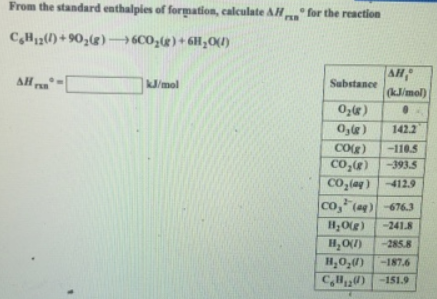# Problem: From the standard enthalpies of formation, calculate ΔHrxn degree for the reaction C6H12 (l) + 9O2 (g) → 6CO2 (g) + H2O (l)

###### FREE Expert Solution
96% (295 ratings)###### Problem Details

From the standard enthalpies of formation, calculate ΔHrxn degree for the reaction

C6H12 (l) + 9O2 (g) → 6CO2 (g) + H2O (l)